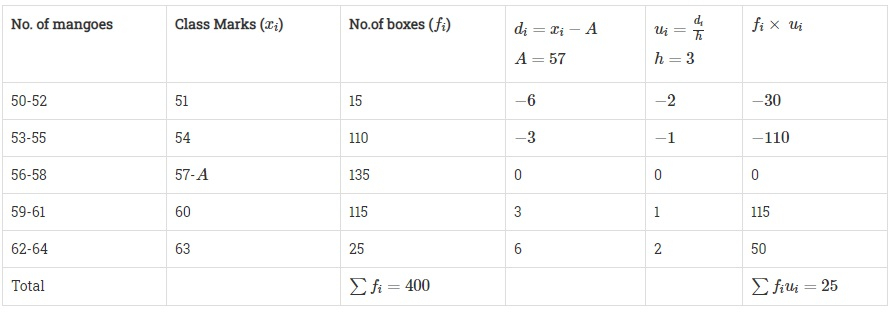# In a retail market, fruit vendors were selling mangoes kept in packing boxes. These boxes contained varying number of mangoes. The following was the distribution of mangoes according to the number of boxes.Number of mangoes:50-5253-5556-5859-6162-64Number of boxes:1511013511525 Find the mean number of mangoes kept in a packing box. Which method of finding the mean did you choose?

Given:

In a retail market, fruit vendors were selling mangoes kept in packing boxes. These boxes contained varying number of mangoes.

The distribution of mangoes according to the number of boxes is given.

To do:

We have to find the mean number of mangoes kept in a packing box.

Solution:

Let the assumed mean be $A = 57$.We know that,

Mean $=A+h \times \frac{\sum{f_iu_i}}{\sum{f_i}}$

Therefore,

Mean $=57+3\times\frac{25}{400}$

$=57+\frac{75}{400}$

$=57+0.19$

$=57.19$

The mean number of mangoes kept in a packing box is $57.19$. We used assumed mean deviation method.

Updated on: 10-Oct-2022

43 Views